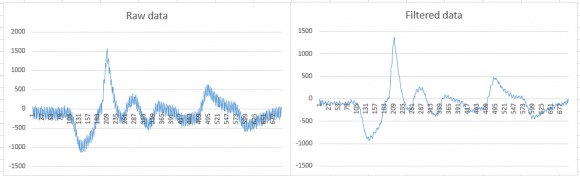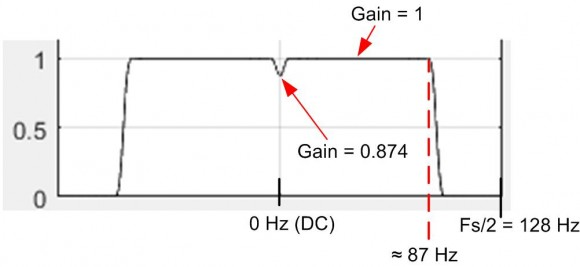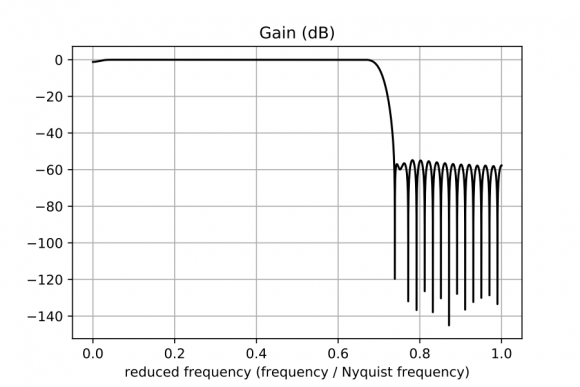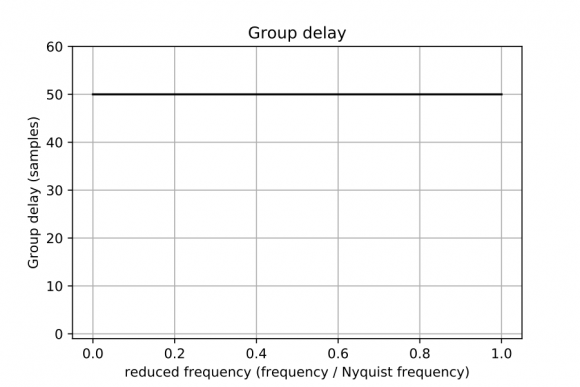## questions of digital filter in a legacy applicationStarted by 4 years ago18 replieslatest reply 4 years ago86 views

I am supporting a legacy application written in C++.  The application includes source code for a digital filter.  I am trying to understand what type of digital filter this is and what its characteristics are.   I am functionally illiterate when it comes to digital filters and the math involved.

I have the original source code (i.e. C++ class) used as a proof of concept.  There are only 139 lines of code in the class and the Wide Open License (WOL) copyright is credited to Grant R. Griffin (2000).  If this might be of any assistance, I have found a number of blog posts by Grant Griffin.  The only information about the filter in the source code is the following comment:

The digital filter used in this application is a FIR (Finite Impulse Response) filter.
The filter is designed using windowing method. Using Hamming window, the minimum stop
band attenuation of the filter is 54 dB and its transition bandwidth is 6.64FN/ N,
where FN is the maximum system frequency and where N is the filter order.

My question is as follows.  Can anyone recommend a forum or newsgroup where I can post the source code for the purposes of determining what type of filter this is and its characteristics (e.g. roll-off and slope)?

thanks,

John

[ - ]Hello John1234567890.

When designing a FIR filter using the "window method" the result of the design process is a sequence of numbers. That sequence of numbers is typically called the "filter coefficients" (some people call that sequence the "filter tap weights").

I can't read C code but you can. Review the code and determine what is the sequence of filter "coefficients" produced by the code. The coefficients will be "symmetrical" in that the first sample in the sequence will be equal to the last sample in the sequence. Likewise, the second sample in the sequence will be equal to the next to the last sample in the sequence, and so on.

If you can post that list of coefficient sample values here, we can determine everything there is to know about the frequency-domain behavior of designed FIR filter.

Also, you may be able to contact Grant Griffin by using the following web page:

[ - ]Hello Rick,

Thank you for the link to Grant Griffins contact page.  Would you prefer I first contact Grant before requesting assistance here?  If so then please let me know and, in this case, ignore the following information.

The next figure shows the graph of the filter coefficients:There are 101 coefficients and they are as follows:

-0.00042 0.000334 -0.00058 -0.00029 0.000359 -0.00089 -0.0001 0.00033 -0.00135 0.000255 0.000123 -0.0019 0.000852 -0.00043 -0.00239 0.001684 -0.00148 -0.00257 0.002624 -0.00313 -0.00215 0.003408 -0.00535 -0.00084 0.003646 -0.00793 0.001561 0.002858 -0.01048 0.005134 0.000525 -0.0124 0.009757 -0.00387 -0.0129 0.015115 -0.01088 -0.01095 0.020724 -0.02127 -0.00498 0.025989 -0.03688 0.008325 0.030301 -0.06444 0.041262 0.033129 -0.15409 0.253095 0.700781 0.253095 -0.15409 0.033129 0.041262 -0.06444 0.030301 0.008325 -0.03688 0.025989 -0.00498 -0.02127 0.020724 -0.01095 -0.01088 0.015115 -0.0129 -0.00387 0.009757 -0.0124 0.000525 0.005134 -0.01048 0.002858 0.001561 -0.00793 0.003646 -0.00084 -0.00535 0.003408 -0.00215 -0.00313 0.002624 -0.00257 -0.00148 0.001684 -0.00239 -0.00043 0.000852 -0.0019 0.000123 0.000255 -0.00135 0.00033 -0.0001 -0.00089 0.000359 -0.00029 -0.00058 0.000334 -0.00042

The next figure shows the unfiltered (left side) and filtered (right side) data.  The input signal has 701 samples with a sampling rate of 256Hz.Thank you for taking the time to respond.

[ - ]Hi.

To add just a little information to Laurent Millot's nice plots, below is your lowpass filter's frequency magnitude response plotted using a linear vertical axis. I noticed something a bit peculiar.Your filter's passband gain up to the 87 Hz "cutoff" frequency is, in general, one (unity) except for a strange "gain depression" dip a zero Hz. At zero Hz the gain is 0.874. I don't recall ever before seeing such a characteristic in a lowpass FIR filter designed using the "window design" method.

[ - ]Hello Rick,

The test code sets the band pass to 0.3Hz to 90Hz.  In addition (and something I should have mentioned), it also sets a notch filter with a (seemingly inverted) low pass setting of 65Hz and a high pass setting of 55Hz.

The comment in the source code states, "transition bandwidth is 6.64FN/ N, where FN is the maximum system frequency and where N is the filter order".  In the sample code, the sampling rate is 256 Hz. So does this mean the maximum system frequency is 128 Hz (i.e. 256/2)?   Similarly, the number of coefficients is set to 101.  So does this mean the filter order is 50 (i.e. 101/2 rounded up to an integer)?  Given the formula of 6.64FN/N, would the value computed for the transition bandwidth be 17 Hz (i.e. 6.64 x 128 / 50)?  Finally, would a value of 17 Hz mean the transition from the passband to the stopband in the frequency response graph occurs within 17 Hz?

What I need is a description of this digital filter that I can include in a user manual.  As such, anyone reading the user manual would understand what/how the digital filter is/implemented.   For example, would the following be an sufficient and detailed enough description?

"The digital filter used is a FIR (Finite Impulse Response) filter. The filter is designed using windowing method. Using Hamming window, the minimum stop band attenuation of the filter is 54 dB and its transition bandwidth is 6.64FN/ N, where FN is half the sampling rate and N is 50.  For example, if the sampling rate is 256 Hz then the transition bandwidth is 17 Hz (i.e. 6.64 x (256/2) / 50)."

Thanks

[ - ]Hi John. The above comment: "...where FN is the maximum system frequency..." is unfortunate because we don't know what the phrase "maximum system frequency" means.

Also, the "FN" notation is non-standard. I've measured the transition region width of your filter to be roughly 8 Hz. As such, I recommend the following text for your User's Manual:

"The digital filter is a linear-phase tapped-delay line FIR (Finite Impulse Response) filter. It is a 100th-order (101 taps) filter designed using the Windowing Design method with a Hamming window. The lowpass filter's passband width is 0.34Fs Hz where Fs is the input data sampling rate. The passband gain is one and the filter's minimum stopband attenuation 54 dB. The filter's transition region width is 6.64Fs/200.  For example, if the the input data sampling rate is 256 Hz then the transition region width is 8.5 Hz (i.e. 6.64 x 256/200)."

For guys who are familiar with FIR filters, my above text tells them what they need to know. There's one piece of missing information that you'll have to supply. That is: I think you should include, in my above paragraph, the numerical nature of the filter coefficients. That is, are the filter's coefficients represented by "floating-point" numbers or "fixed-point binary" numbers? If the coefficients are represented by "fixed-point binary" numbers then you should specify how many binary bits are used to represent a coefficient value.

John, regarding your above 1st paragraph discussing "...it also sets a notch filter with a (seemingly inverted) low pass setting of 65Hz and a high pass setting of 55Hz." confuses the daylights out of me. We have to figure out what those strange words mean.

[ - ]Hello Rick,

1) Thank you for that description. I will add this to the user manual and it will buy me some time to sit down and learn the basics about digital filters.

1a) Your description includes the divisor 200 (i.e. 64Fs/200). Is the number 200 determined by the filter order of 100? In other words, if the filter order was changed to say 25 (i.e. 26 'taps') then would the divisor be 50, that is, twice the filter order?

2) You asked, "are the filter's coefficients represented by "floating-point" numbers or "fixed-point binary" numbers?". The answer is they are represented by "floating point" (i.e. double precision) numbers.

3) You wrote, "...confuses the daylights out of me". The sample source code applies a notch filter to the input data and sets the high pass filter setting to 65Hz and the low pass filter setting to 55Hz. I was expecting the reverse (i.e. the high pass to be 55 and the low pass to be 65). The mistake is mine and what I should have written instead is:

...it also sets a notch filter with a (seemingly inverted)low high pass setting of 65Hz and a high low pass setting of 55Hz.
[ - ]Hi John.

Answer# 1a): You wrote that "the source code states, transition bandwidth is 6.64FN/ N, where FN is the maximum system frequency and where N is the filter order." I don't know the origin of that simple formula but I'll assume it's correct. Also, the "order" of your tapped-delay line FIR filter is N = 100 (equal to the number of coefficients minus one). If I assume that "maximum system frequency" means Fs/2 = 128 Hz then the formula states that the transition region width will be 6.64*(128)/100 = 8.49 Hz, which is very close to my measured value of your filter's the transition region width.

If N is changed to 25 (your 26 coefficients minus one) then that formula (that we've decided to trust) says the transition region width will be 6.64*128/25 = 34 Hz. DSP guru fred harris (who regularly posts replies in this Forum) developed a useful rule of thumb formula stating that for a lowpass FIR filter with a reasonably flat passband and a fixed stopband attenuation, the filter's transition region width is roughly inversely proportional to the number of filter coefficients. (If you halve the number of coefficients, the transition region width will roughly double.) I cover this topic in more detail at the end of Chapter 5 in my book.

Answer# 3): I don't know anything about a notch filter. *ALL* of my comments here are based solely on the 101 coefficient values you posted on Feb. 11, 2019 for a lowpass FIR filter. The frequency magnitude response of a FIR filter using your 101 coefficients has no notch in the vicinity of 60 Hz.

[ - ]Hello John,

Your filter is a linear phase FIR lowpass filter:

- FIR: finite impulse response;

- linear phase: the phase plot gives a line for most of the frequencies (maybe the coefficients given in your last post are truncated) so the group delay is a constant;

- a linear phase or constant group delay indicates that the input order for the frequency components is conserved at the output which means that you filter does not modify the phase and just alters the amplitude of each frequency component according to the chosen gain plot.

One of the linear phase filters characteristics is that the impulse response is symmetrical or anti-symmetrical when considering its time center: here the sample #50.

You will find enclosed the python 3.5 script and the plots which illustrate my proposal.

Laurent Millot

determine_filter_nature.py[ - ]Hello Laurent,

I have not dealt with digital filters before.  I have to acknowledge I really do not know how to interpret the graphs you provided.  I feel like a fish out of water.  So I spent a few hours looking for books today and see Rick actually has one on Amazon with a great rating of about 4.5/5.   I ordered it.

Thanks for taking the time to help out.

John
[ - ]Hi John. If you have a copy of my "Understanding DSP" book. Start by reading Chapters 1 & 2, and then go to Chapter 5. Let me know if you have any questions <R_dot_Lyons_@_ieee_dot_org>.

[ - ]Hello Rick,

Your book will be delivered to me in a few days and I will read these chapters.

John

[ - ]Hi John. I forgot to say, after the book arrives visit the following web page, scroll down to the "AMERICAN 3RD EDITION", and click on the words: "Errata 3rd Ed. 6th and above Printings.pdf"

Good Luck.

[ - ]Hello Rick,

If you don't mind, I have two last questions.

1. Your description includes the divisor 200 (i.e. 64Fs/200). Is the number 200 determined by the filter order of 100? In other words, if the filter order was changed to say 25 (i.e. 26 'taps') then would the divisor be 50, that is, twice the filter order?
2.  Does the number of filter coefficients determine the effectiveness of the filter (i.e. the extent to which frequencies outside the passband are removed from the input signal).  For example, will 50 coefficients (i.e. half of 101) mean the filter is 'less effective' while 202 coefficients (i.e. two times 101) mean the filter is 'more effective'? In other words, does changing the number of coefficients change the description of the filter that you provided?

Thank you so much for all of your time and patience.

John

[ - ]Hi John.

Answer# 1: I prefer to write that equation as:

Trans. Reg. Width = 6.64*(Fs/2)/N Hz

where the variable 'N' is dimensionless. When Fs = 256 Hz and the filter order is N = 25 (26 taps) the equation becomes:

Trans. Reg. Width = 6.64*(128)/25 = 34 Hz.

Answer# 2: Because the word "effectiveness" is somewhat vague, I prefer to use the word "performance". And the answer to your question is that the number of FIR filter taps directly and profoundly affects the frequency-domain performance of the filter. For me, high stopband attenuation means high performance. Likewise, a narrow transition region width also means high performance. Based on modern FIR filter design software, the more taps in a tapped-delay line FIR filter, designed with a given transition region width, the higher will be the stopband attenuation. Similarly, the more taps in a tapped-delay line FIR filter, designed with a given stopband attenuation, the more narrow will be the transition region width.

You wrote: " Thank you so much for all of your time and patience." I'm happy to help if I am able. Who knows, perhaps some day when you're rich & famous you'll thank me by sending me one of the Human Race's greatest creations; a pack of Hostess orange cupcakes.
[ - ]Hi John,

Indeed, to understand the plots you need to study "a little" at least digital signal processing...

If you encounter some difficulties with Rick's book (I haven't read this book for the moment) you may have a look to Smith's one (the scientist ans engineer's guide to digital signal processing) where the mathematical difficulties are rather reduced.

There are also the Julius Orion Smith III books but there are plenty of mathematical formulas...

In fact, if you really want to understand signal processing, you must try all you can using programming environments and signal libraries: Matlab or Python if you know programmation; Max (former Max/MSP) can help with its graphical programming (Pure Data used to be an alternative). Applying signal processing even for rather simple filters is one major key.

Using audio devices, softwares or even Digital Audio Workstation can also help but you need to listen how to manage them but, most of the time, you are in front of a "black box".

This is why I do prefer to use not so expensive solutions to test ideally everything in parallel when trying to learn signal processing. Python is free. Max costs a little if you intend to re-use it after your learning phase. Pure Data is free but the main project has been abandoned and the "revival" projects may be considered carefully for the moment.

I used Matlab and Pure Data for more than 20 years, either for musical acoustics and audio processing, but I adopted Python programming three years ago (to be able to export rather easily either packages or even stand-alone applications)and Max last summer.

Laurent Millot

[ - ]Hello Laurent,

I am halfway through chapter 2 in Rick's book and am digesting the concepts and principles introduced so far.  The math in later chapters will be a problem for me.   So for now, my goal is simply to understand basic concepts and terms.

Thanks for the reference to books by Steven Smith.  I know they are available online on this site.

I am comfortable working with Matlab.  I expect the only issue with Python is actually finding the time to learn it along with it's libraries.  I will take a look at the other software tools you recommend as I make progress.

Thank you,

John

[ - ]HI John.

My book has a bit more mathematics that Steven Smith's terrific book, but please know that I wrote my book to keep the mathematics at a low -to- moderate level to make it more understandable than the typical college DSP textbook. If you encounter an equation in my book that's not explained clearly enough for you, please let me know.

[ - ]